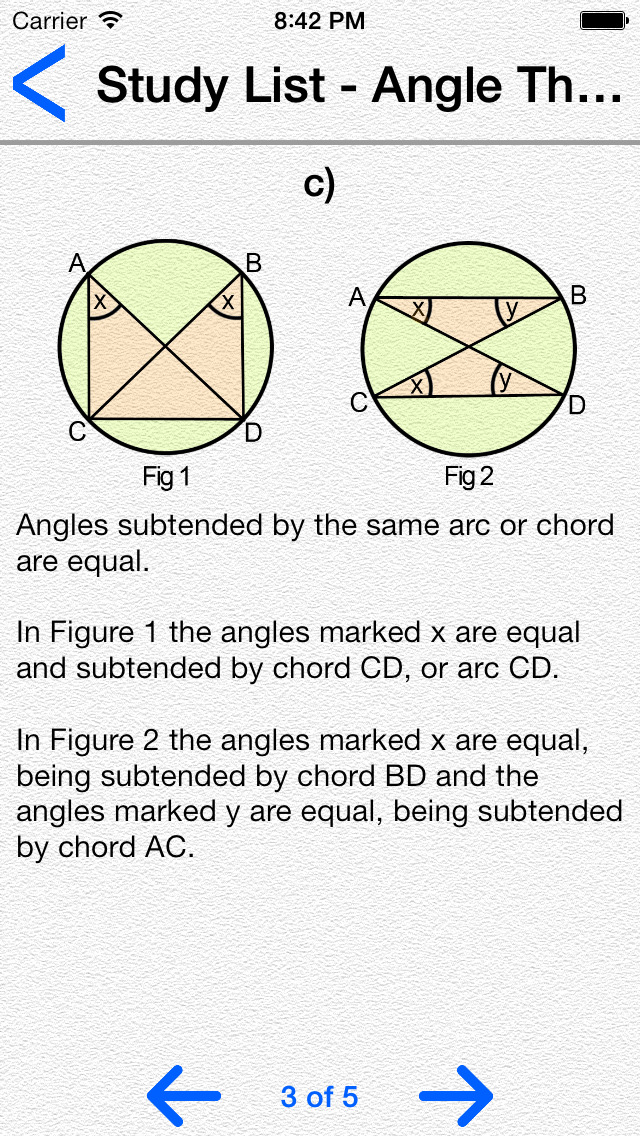# Math Yes Or No

## iOS UniversalEducation

Free

Can you plus, minus, multiply and divid. Compete with the whole world in Game Center, and become Math a champion.

- Features
- 6 different game types
- Hundreds of interesting questions
- Game center
- Achievements
- Universal App (iPhone, iPod, iPad) buy once use in all devices.

Detailed Explanation on Below Topics.

--- Number ---
- Decimals
- Fractions
- Number Sequences
- Surds
- Percentages
- Positive & Negative Numbers
- Standard Form
- Ratios
- Proportion

--- Shape and Space ---
- Angle Theorems
- Angles
- Circle Theorem
- Shapes, Symmetry & Tesselation
- Loci
- Areas and Volumes
- Ruler and Compass Constructions
- Vectors
- Transformations

--- Statistics ---
- Probability
- Averages
- Standard Deviation
- Sampling
- Cumulative Frequency Graphs
- Representing Data
- Histograms

--- Algebra ---
- Factorising
- Solving Equations
- Travel Graphs
- Algebraic Fractions
- Equation of a Straight Line
- Simultaneous Equations
- Inequalities
- Indices
- Functions

--- Trigonometry ---
- Sin, Cos, Tan
- Pythagoras
- Sine and Cosine Rule
- Bearings
- Similar Triangles
- Congruency

## What's New

- Optimized for iPhone 6 and 6-plus

•••••